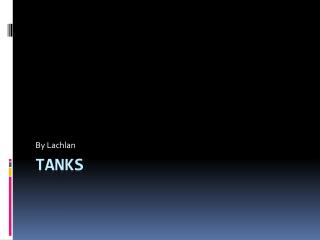DownloadDownload PresentationTanks

# Tanks

Télécharger la présentation## Tanks

- - - - - - - - - - - - - - - - - - - - - - - - - - - E N D - - - - - - - - - - - - - - - - - - - - - - - - - - -
##### Presentation Transcript

1. By Lachlan Tanks

2. M60-A3 Main Battle Tank • This tanks fastest is 48km/h • The length is 6.946 excluding gun • The width is 3.631 • The height is 3.27m • The armour max is 120mm ( estimated) • The crew is four

3. Chieftain Mk X Main Battle Tank • The length is 7.518m • The Width is 3.504m • It weights 55,000kg • The Crew is four • The max road speed is 48kph

4. Centurion Main Battle Tank • The length is 7.823m • the Width is 3.39m • It weights 51,820kg • The crew is four • Max road speed is 34.5kph

5. T-55 M • The length is 6.45m • The width is 3.57m • The weight is 36,000kg • The crew is four • The Max road speed 48 kph

6. Daimler Ferret Scout Car • Length 3.835m • The width is 1.905m • The crew is two • The max road speed is 93 kph

7. Alvis Scorpion (FV101) • The length is 4.794m • The width is 2.235m • The crew is three • The max road speed is 80.5 kph

8. Alvis Saladin armoured car FV601 • The length is 4.93m • the width is 2.54m • Its weight is 11,590kg • Its crew is three • The max road speed is 72 kph

9. scimitar • The length is 4.79m • The width is 2.235m • The weight is 8,073kg • The crew is three • The top road speed is 80.5 kph

10. Alvis Saracen APC • The length is 5.251 • The width is 2.8m • The weight is15,280kg • The crew is 2+10 • The max road speed is 52.2 kph